# 5 Free Books to Learn Statistics for Data Science

Learn all the statistics you need for data science for free.

By Rebecca Vickery, Data ScientistPhoto by Daniel Schludi on Unsplash

Statistics is a fundamental skill that data scientists use every day. It is the branch of mathematics that allows us to collect, describe, interpret, visualise, and make inferences about data. Data scientists will use it for data analysis, experiment design, and statistical modelling.

Statistics is also essential for machine learning. We will use statistics to understand the data prior to training a model. When we take samples of data for training and testing our models we need to employ statistical techniques to ensure fairness. When evaluating the performance of a model we need statistics to assess the variability of the predictions and assess accuracy.

“If statistics are boring, you’ve got the wrong numbers.”, Edward Tufte

These are just some of the ways in which statistics are employed by data scientists. If you are studying data science it is therefore essential to develop a good understanding of these statistical techniques.

This is one area where books can be a particularly useful study tool as detailed explanations of statistical concepts is essential to your understanding.

Here are my top 5 free books for learning statistics for data science.

### Practical Statistics for Data Scientists

by Peter Bruce and Andrew Bruce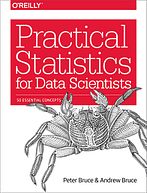Image: amazon.co.uk

Main topics covered:

• Data structures.
• Descriptive statistics.
• Probability.
• Machine learning.

Suitable for: Complete beginners.

Statistics is a very broad field, and only part of it is relevant to data science. This book is extremely good at only covering the areas related to data science. So if you are looking for a book that will quickly give you just enough understanding to be able to practice data science then this book is definitely the one to choose.

It is filled with a lot of practical coded examples (written in R), gives very clear explanations for any statistical terms used and also links out to other resources for further reading.

This is overall an excellent book to cover off the basics and is suitable for an absolute beginner to the field.

### Think Stats

by Allen B. Downey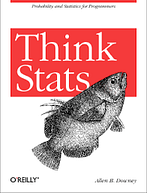Main topics covered:

• Statistical thinking.
• Distributions.
• Hypothesis testing.
• Correlation.

Suitable for: Beginners with basic Python.

The introduction for this book states that “this book is about turning knowledge into data” and it does a very good job of introducing statistical concepts through practical examples of data analysis.

“this book is about turning knowledge into data”

It is another book that covers only the concepts directly related to data science and also contains lots of code examples, this time written in Python. It is aimed heavily at programmers and relies on using that skill to understand the key statistical concepts introduced. This book is therefore ideally suited to those who already have at least a basic grasp of Python.

### Bayesian Methods for Hackers

by Cameron Davidson-Pilon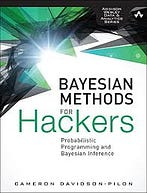Image: amazon.com

Main topics covered:

• Bayesian inference.
• Loss functions.
• Bayesian machine learning.
• Priors.

Suitable for: Non-statisticians with a working knowledge of Python.

Bayesian inference is a branch of statistics that deals with understanding uncertainty. As a data scientist uncertainty is something you will need to model on a very regular basis. If you are building a machine learning model, for example, you will need to be able to understand the uncertainty around the predictions that your model is delivering.

Bayesian methods can be quite abstract and difficult to understand. This book aimed firmly at programmers (so some Python is a prerequisite), is the only material I have found that explains these concepts in a simple enough way for a non-statistician to understand. There are coded examples throughout and the Github repository, where the chapters are hosted, contains a large selection of notebooks. It is, therefore, an excellent hands-on introduction to this subject.

### Statistics in Plain English

by Timothy C. Urdan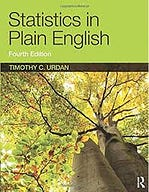Image: amazon.co.uk

Main topics covered:

• Regression.
• Distributions.
• Factor analysis.
• Probability.

Suitable for: Non-statisticians with any level of programming experience.

This book covers general statistical techniques rather than just those aimed at data scientists or programmers. It is however written in a very straight forward style and covers a wide range and depth of statistical concepts in a very simple to understand way.

The book was originally written for students studying a non-mathematics based course where an understanding of statistics is required, such as the social sciences. It, therefore, covers enough theory to understand the techniques but doesn’t assume an existing mathematical background. It is, therefore, an ideal book to read if you are coming into data science without a math-based degree.

### Computer Age Statistical Inference

by Bradley Efron and Trevor Hastie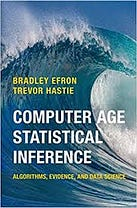Image: amazon.co.uk

Main topics covered:

• Bayesian and frequentist inference.
• Large scale hypothesis testing.
• Machine learning.
• Deep learning.

Suitable for: Someone with a basic understanding of statistics and statistical notation. No programming required.

This book covers the theory behind most of the popular machine learning algorithms used by data scientists today. It also gives a thorough introduction to both Bayesian and Frequentist statistical inference methodologies.

The second half of the book, which covers machine learning algorithms, is some of the best material I have seen on this subject. Each explanation is in-depth and uses practical examples such as the classification of spam data which makes quite complex ideas easier to digest. The book is most suited to those who have already covered the basics of statistics for data analysis and are familiar with some statistical notation.

The books I included in this article cover enough topics for a complete beginner to learn all the statistics needed for data science. They can all be read for free online but most also have a print version that can be purchased if you prefer to read physical books. Statistics is an essential component of the data science toolset and something which often requires in-depth reading to truly understand the concepts. Something which these books can provide.

For more data science reading lists please check out my previous articles below.

5 Free Books for Learning Python for Data Science
A completely free reading list for learning Python

Completely Free Machine Learning Reading List
10 free books to read if you are studying machine learning

Reading List for Applied AI
Six books to read if you are applying AI to your business in 2020

I send out a monthly newsletter if you would like to join please sign up via this link. Looking forward to being part of your learning journey!

Bio: Rebecca Vickery is learning data science through self study. Data Scientist @ Holiday Extras. Co-Founder of alGo.

Original. Reposted with permission.

Related:

By subscribing you accept KDnuggets Privacy Policy

By subscribing you accept KDnuggets Privacy Policy

By subscribing you accept KDnuggets Privacy Policy